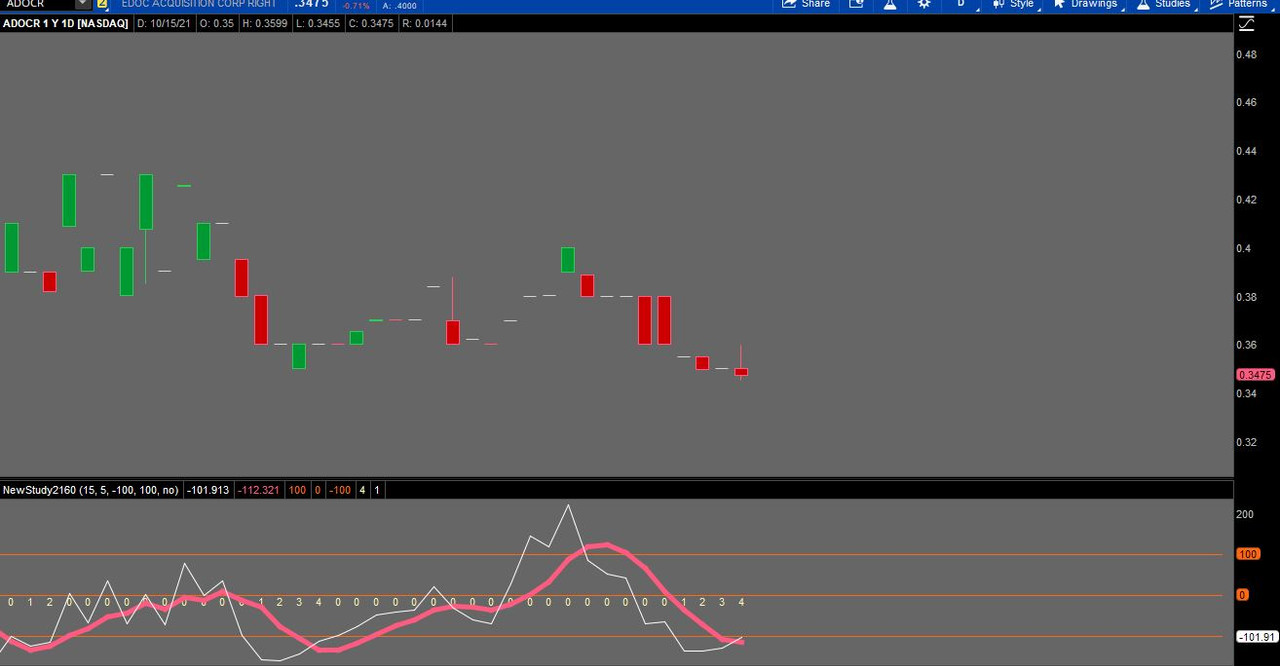# How to turn indicator into scanner as follows

#### c0der

##### Member
I want the scanner to flag when

CCI15<-100 in last 3 bars
CCI15 cross ABOVE CCI15(MA 5) Signal Line in last bar

My indicator code is below. How do I turn it into a scanner?

Code:
``````declare lower;

input length = 15;
input avglength = 5;
input over_sold = -100;
input over_bought = 100;
input showBreakoutSignals = no;

def price = close + low + high;
def linDev = lindev(price, length);
plot CCI = if linDev == 0 then 0 else (price - Average(price, length)) / linDev / 0.015;
plot CCI_avg = Average(CCI, avglength);
plot OverBought = over_bought;
plot ZeroLine = 0;
plot OverSold = over_sold;
plot UpSignal = if CCI crosses above ZeroLine then ZeroLine else Double.Nan;
plot DownSignal = if CCI crosses below ZeroLine then ZeroLine else Double.Nan;

UpSignal.SetHiding(!showBreakoutSignals);
DownSignal.SetHiding(!showBreakoutSignals);

CCI.setDefaultColor(GetColor(9));
OverBought.setDefaultColor(GetColor(5));
ZeroLine.setDefaultColor(GetColor(5));
OverSold.setDefaultColor(GetColor(5));
UpSignal.SetDefaultColor(Color.UPTICK);
UpSignal.SetPaintingStrategy(PaintingStrategy.ARROW_UP);
DownSignal.SetDefaultColor(Color.DOWNTICK);
DownSignal.SetPaintingStrategy(PaintingStrategy.ARROW_DOWN);``````

Solution
still looking for a solution.
This is the code for the scanner
Ruby:
``````declare lower;

input length = 15;
input avglength = 5;
input over_sold = -100;
input over_bought = 100;
input showBreakoutSignals = no;

def price = close + low + high;
def linDev = LinDev(price, length);
def CCI = if linDev == 0 then 0 else (price - Average(price, length)) / linDev / 0.015;
def CCI_avg = Average(CCI, avglength);
def OverBought = over_bought;
def ZeroLine = 0;
def OverSold = over_sold;
def UpSignal = if CCI crosses above ZeroLine then ZeroLine else Double.NaN;
def DownSignal = if CCI crosses below ZeroLine then ZeroLine else Double.NaN;

#UpSignal.SetHiding(!showBreakoutSignals);
#DownSignal.SetHiding(!showBreakoutSignals)...``````

#### SleepyZ

##### Well-known member
still looking for a solution.
This is the code for the scanner
Ruby:
``````declare lower;

input length = 15;
input avglength = 5;
input over_sold = -100;
input over_bought = 100;
input showBreakoutSignals = no;

def price = close + low + high;
def linDev = LinDev(price, length);
def CCI = if linDev == 0 then 0 else (price - Average(price, length)) / linDev / 0.015;
def CCI_avg = Average(CCI, avglength);
def OverBought = over_bought;
def ZeroLine = 0;
def OverSold = over_sold;
def UpSignal = if CCI crosses above ZeroLine then ZeroLine else Double.NaN;
def DownSignal = if CCI crosses below ZeroLine then ZeroLine else Double.NaN;

#UpSignal.SetHiding(!showBreakoutSignals);
#DownSignal.SetHiding(!showBreakoutSignals);

#CCI.SetDefaultColor(GetColor(9));
#OverBought.SetDefaultColor(GetColor(5));
#ZeroLine.SetDefaultColor(GetColor(5));
#OverSold.SetDefaultColor(GetColor(5));
#UpSignal.SetDefaultColor(Color.UPTICK);
#UpSignal.SetPaintingStrategy(PaintingStrategy.ARROW_UP);
#DownSignal.SetDefaultColor(Color.DOWNTICK);
#DownSignal.SetPaintingStrategy(PaintingStrategy.ARROW_DOWN);

def cci15minus100 = if CCI crosses below -100 then 1 else if cci > -100 then 0 else cci15minus100 + 1;
plot scan = if cci15minus100 >= 3 and CCI crosses above CCI_avg then 1 else 0;``````

This is the code for the chart to test resultsRuby:
``````declare lower;

input length = 15;
input avglength = 5;
input over_sold = -100;
input over_bought = 100;
input showBreakoutSignals = no;

def price = close + low + high;
def linDev = lindev(price, length);
plot CCI = if linDev == 0 then 0 else (price - Average(price, length)) / linDev / 0.015;
plot CCI_avg = Average(CCI, avglength);
plot OverBought = over_bought;
plot ZeroLine = 0;
plot OverSold = over_sold;
plot UpSignal = if CCI crosses above ZeroLine then ZeroLine else Double.Nan;
plot DownSignal = if CCI crosses below ZeroLine then ZeroLine else Double.Nan;

UpSignal.SetHiding(!showBreakoutSignals);
DownSignal.SetHiding(!showBreakoutSignals);

CCI.setDefaultColor(GetColor(9));
OverBought.setDefaultColor(GetColor(5));
ZeroLine.setDefaultColor(GetColor(5));
OverSold.setDefaultColor(GetColor(5));
UpSignal.SetDefaultColor(Color.UPTICK);
UpSignal.SetPaintingStrategy(PaintingStrategy.ARROW_UP);
DownSignal.SetDefaultColor(Color.DOWNTICK);
DownSignal.SetPaintingStrategy(PaintingStrategy.ARROW_DOWN);

def cci15minus100 = if cci crosses below -100 then 1 else if cci > -100 then 0  else cci15minus100+1;
plot cci15minus100_ =  cci15minus100;
cci15minus100_.setpaintingStrategy(paintingStrategy.VALUES_BELOW);
plot scan = if cci15minus100>=3 and cci crosses above cci_avg then 1 else 0;``````

#### c0der

##### Member
Thank you! Seems unreal that the scanner only gave 1 stock. will try again tomorrow and see how it goes. cheers.